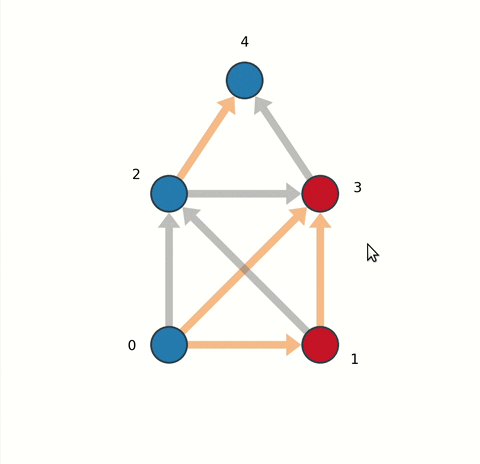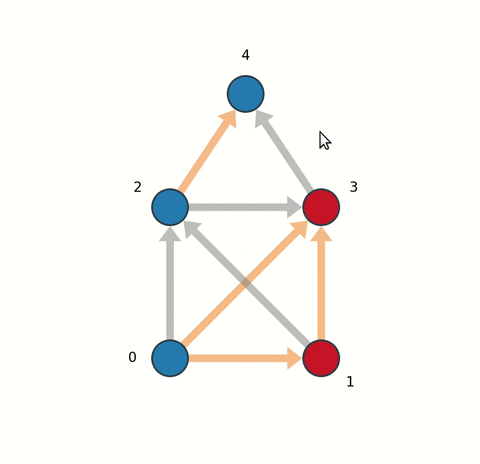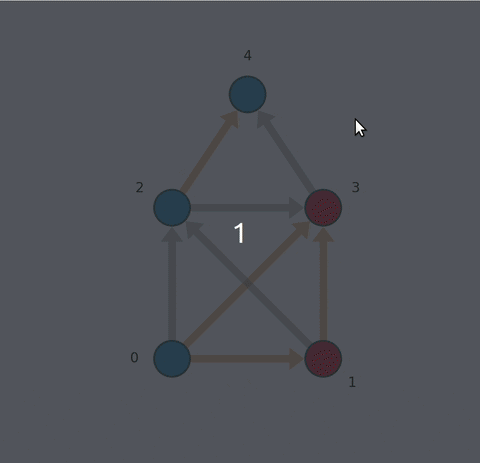Need help with netgraph?
Click the “chat” button below for chat support from the developer who created it, or find similar developers for support.paulbrodersen289 Stars24 ForksGNU General Public License v3.0547 Commits2 Opened issues#### Description

Drawing utilities for publication quality plots of networks!
?

#### Need anything else?#### Contributors list# 239,390
Shell
Jupyter...
C
simulat...
512 commits# 170,575
Vue.js
hot-rel...
Webpack
HTML
2 commits# 180,714
indoor-...
TeX
dropbox
diary-a...
2 commits# 429,111
Python
Tensorf...
visuali...
network...
1 commit

# netgraph

Python drawing utilities for publication quality plots of networks.

### Quickstart

Install with:

```pip install netgraph
```

Import module and plot with:

```import numpy as np
import matplotlib.pyplot as plt
from netgraph import Graph, InteractiveGraph, EditableGraph

Several graph formats are supported:
graph_data = [(0, 1), (1, 2), (2, 0)] # edge list
graph_data = [(0, 1, 0.2), (1, 2, -0.4), (2, 0, 0.7)] # edge list with weights
graph_data = np.random.rand(10, 10) # full rank matrix
graph_data = networkx.karate_club_graph() # networkx Graph/DiGraph objects
graph_data = igraph.Graph.Famous('Zachary') # igraph Graph objects
Create a non-interactive plot:
Graph(graph_data)
plt.show()
Create an interactive plot.
NOTE: you must retain a reference to the plot instance!
Otherwise, the plot instance will be garbage collected after the initial draw
and you won't be able to move the plot elements around.
For similar reasons, if you are using PyCharm, you have to execute the code in
a console (Alt+Shift+E).
plot_instance = InteractiveGraph(graph_data)
plt.show()
Create an editable plot.
plot_instance = EditableGraph(graph_data)
plt.show()
help(Graph)
help(InteractiveGraph)
help(EditableGraph)
```

## Reasons why you might want to use netgraph

### Better layouts### Interactivity

Algorithmically finding a visually pleasing graph layout is hard. This is demonstrated by the plethora of different algorithms in use (if graph layout was a solved problem, there would only be one algorithm). To ameliorate this problem, this module contains an

`InteractiveGraph`
class, which allows node positions to be tweaked with the mouse after an initial draw.
• Individual nodes and edges can be selected using the left-click.
• Multiple nodes and or edges can be selected by holding
`control`
while clicking, or by using the rectangle/window selector.
• Selected plot elements can be dragged around by holding left-click on a selected artist.```import matplotlib.pyplot as plt
import networkx as nx
from netgraph import InteractiveGraph

g = nx.house_x_graph()
edge_color = dict()
for ii, edge in enumerate(g.edges):
edge_color[edge] = 'tab:gray' if ii%2 else 'tab:orange'
node_color = dict()
for node in g.nodes:
node_color[node] = 'tab:red' if node%2 else 'tab:blue'
plot_instance = InteractiveGraph(
g, node_size=5, node_color=node_color,
node_labels=True, node_label_offset=0.1, node_label_fontdict=dict(size=20),
edge_color=edge_color, edge_width=2,
arrows=True, ax=ax)
plt.show()
```

There is also some experimental support for editing the graph elements interactively using the

`EditableGraph`
class.
• Pressing
`insert`
or
`+`
will add a new node to the graph.
• Double clicking on two nodes successively will create an edge between them.
• Pressing
`delete`
or
`-`
will remove selected nodes and edges.
• Pressing
`@`
will reverse the direction of selected edges.

When adding a new node, the properties of the last selected node will be used to style the node artist. Ditto for edges. If no node or edge has been previously selected, the first created node or edge artist will be used.Finally, elements of the graph can be labeled and annotated. Labels remain always visible, whereas annotations can be toggled on and off by clicking on the corresponding node or edge.

• To create or edit a node or edge label, select the node (or edge) artist, press the
`enter`
key, and type.
• To create or edit an annotation, select the node (or edge) artist, press
`alt + enter`
, and type.
• Terminate either action by pressing
`enter`
or
`alt + enter`
a second time.```import matplotlib.pyplot as plt
import networkx as nx
from netgraph import EditableGraph

g = nx.house_x_graph()
edge_color = dict()
for ii, (source, target) in enumerate(g.edges):
edge_color[(source, target)] = 'tab:gray' if ii%2 else 'tab:orange'
node_color = dict()
for node in g.nodes:
node_color[node] = 'tab:red' if node%2 else 'tab:blue'
annotations = {
4 : 'This is the representation of a node.',
(0, 1) : dict(s='This is not a node.', color='red')
}
fig, ax = plt.subplots(figsize=(10, 10))
plot_instance = EditableGraph(
g, node_color=node_color, node_size=5,
node_labels=True, node_label_offset=0.1, node_label_fontdict=dict(size=20),
edge_color=edge_color, edge_width=2,
annotations=annotations, annotation_fontdict = dict(color='blue', fontsize=15),
arrows=True, ax=ax)
plt.show()
```

### Exquisite control over plot elements

High quality figures require fine control over plot elements. To that end, all node artist and edge artist properties can be specified in three ways:

1. Using a single scalar or string that will be applied to all artists.
```import matplotlib.pyplot as plt
from netgraph import Graph

edges = [(0, 1), (1, 1)]
Graph(edges, node_color='red', node_size=4.)
plt.show()
```

1. Using a dictionary mapping individual nodes or individual edges to a property:
```import matplotlib.pyplot as plt
from netgraph import Graph

Graph([(0, 1), (1, 2), (2, 0)],
edge_color={(0, 1) : 'g', (1, 2) : 'lightblue', (2, 0) : np.array([1, 0, 0])},
node_size={0 : 20, 1 : 4.2, 2 : np.pi},
)
plt.show()
```

1. By directly manipulating the node and edge artists (which are derived from matplotlib PathPatch artists):
```import matplotlib.pyplot as plt; plt.ion()
from netgraph import Graph

fig, ax = plt.subplots()
g = Graph([(0, 1), (1, 2), (2, 0)], ax=ax)
make some changes
g.edge_artists[(0, 1)].set_facecolor('red')
g.edge_artists[(1, 2)].set_facecolor('lightblue')
force redraw to display changes
fig.canvas.draw()
```

Similarly, node and edge labels are just matplotlib text objects. Their properties can also be specified using a single value that is applied to all of them:

```import matplotlib.pyplot as plt
from netgraph import Graph

Graph([(0, 1)],
node_size=20,
node_labels={0 : 'Lorem', 1 : 'ipsum'},
node_label_fontdict=dict(size=18, fontfamily='Arial', fontweight='bold'),
edge_labels={(0, 1) : 'dolor sit'},
# blue bounding box with red edge:
edge_label_fontdict=dict(bbox=dict(boxstyle='round',
ec=(1.0, 0.0, 0.0),
fc=(0.5, 0.5, 1.0))),
)
plt.show()
```

Alternatively, their properties can be manipulated individually after an initial draw:

```import matplotlib.pyplot as plt
from netgraph import Graph

fig, ax = plt.subplots()
g = Graph([(0, 1)],
node_size=20,
node_labels={0 : 'Lorem', 1 : 'ipsum'},
edge_labels={(0, 1) : 'dolor sit'},
ax=ax
)
make some changes
g.node_label_artists.set_color('hotpink')
g.edge_label_artists[(0, 1)].set_style('italic')
force redraw to display changes
fig.canvas.draw()
plt.show()
```

For a full list of available arguments, please consult the docstrings of the

`Graph`
or
`InteractiveGraph`
class:
```from netgraph import Graph; help(Graph)
```

### Consistent length units

Existing drawing routines for networks in python (networkx, igraph) use fundamentally different length units for different plot elements. For example, networkx uses data units to specify node positions but display units for node sizes. This makes it difficult to judge the relative sizes of plot elements a priori. Also, layouts cannot be exactly reproduced on different computers, if their display sizes differ.

This module amends these issues by having a single reference frame that derives from the data. Specifically, node positions and edge paths are specified in data units, and node sizes and edge widths are given in 1/100 of data units (i.e. a node with

`node_size=2`
has a radius of 0.02 in data units). Rescaling by 1/100 makes the node sizes and edge widths more comparable to typical node sizes in igraph and networkx.

### Compatibility with igraph and networkx

Many people that analyse networks in python use several network analysis libraries, e.g. igraph and networkx. To facilitate interoperability, various network formats are supported:

1. Edge lists:

Iterable of (source, target) or (source, target, weight) tuples, or equivalent (m, 2) or (m, 3) ndarray.

Full-rank (n, n) ndarray, where n corresponds to the number of nodes. The absence of a connection is indicated by a zero.

1. igraph.Graph or networkx.Graph objects

## Help, I don't know how to do ...!

Please raise an issue. Include any relevant code and data in a minimal, reproducible example. If applicable, make a sketch of the desired result with pen and paper, take a picture, and append it to the issue.

Bug reports are, of course, always welcome. Please make sure to include the full error trace.

If you submit a pull request that fixes a bug or implements a cool feature, I will probably worship the ground you walk on for the rest of the week. Probably.

Finally, if you do email me, please be very patient. I rarely check the email account linked to my open source code, so I probably will not see your emails for several weeks, potentially longer. Also, I have a job that I love and that pays my bills, and thus takes priority. That being said, the blue little notification dot on github is surprisingly effective at getting my attention. So please just raise an issue.